# Optical properties of materials¶

Solcore has several ways of accessing the optical properties of materials: databases and parametric dielectric functions.

## Dielectric constants models and Adachi parametrization¶

Understanding the optical response of both established and novel materials is crucial to effective solar cell design. To efficiently model the complex dielectric function of a material Solcore incorporates an optical constant calculator based on the well-known Critical-Point Parabolic-Band (CPPB) formalism popularised by Adachi (, , ). In this model, contributions to :math:epsilon_2(omega) from critical points in the Brillouin Zone at which the probability for optical transitions is large (van Hove singularities) are considered. The transition probability for such transitions is proportional to the joint density of states (JDOS)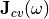, which describes the number of available electronic states between the valence and conduction bands at given photon energy. The imaginary part of the complex dielectric function is related to the JDOS by: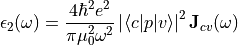Where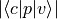is the momentum matrix element for transitions from the valence band (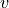) to the conduction band (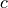). Critical point transitions are considered at the following points of symmetry in the band structure: :math:E_0 corresponds to the optical transition at the :math:Gamma point and :math:E_0 + Delta_0 to the transition from the spin-orbit split off band to the conduction band at the :math:Gamma point. :math:E_1 and :math:E_1 + Delta_1 denote the transitions from the valence heavy-hole (HH) band and the valence light-hole (LH) band respectively to the conduction band at the L point. The :math:E’_0 triplet and :math:E_2 transitions occur at higher energies, between the HH band and the split conduction bands at the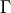point as well as across the wide gap X valley. The model also includes contributions from the lowest energy indirect band-gap transition and the exciton absorption at the :math:E_0 critical point. The contributions listed above are summed to compute the overall value of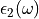. The real and imaginary components of the overall complex dielectric function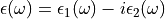are then related via the Kramers-Kronig relations;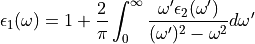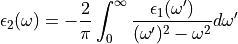The CPPB model included with Solcore also incorporates a modification to the critical point broadening present in Adachi’s description, which is shown to produce a poor fit to experimental data in the vicinity of the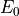and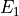critical points (). To give a more accurate description of the broadening of the optical dielectric function, Kim et al. proposed that a frequency-dependent damping parameter be used to replace the damping constant given by Adachi at each critical point (, );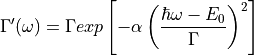Whereis the damping constant used by Adachi and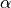describes the shape of the lineshape broadening with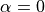producing purely Lorentzian character and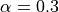producing a good approximation to Gaussian broadening.

The Solcore module absorption_calculator contains the CPPB model within the Custom_CPPB class. The class offers a flexible way to build up the optical constant model by adding individual critical point contributions through the Oscillator structure type within Solcore. In addition to the oscillator functions described by Adachi the Custom_CPPB class also provides additional oscilator models and the Sellmeier equation for describing the real part of the dielectric function for non-absorbing materials ().

Description of functions in this module

## SOPRA database¶

n order to calculate and model the optical response of potential solar cell architectures and material systems, access to a library of accurate optical constant data is essential. Therefore, Solcore incorporates a resource of freely available optical constant data measured by Sopra S. A. and provided by Software Spectra Inc. The refractive index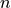and extinction coefficient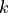are provided for over 200 materials, including many III-V, II-VI and group IV compounds in addition to a range of common metals, glasses and dielectrics.

Any material within the Sopra S.A. optical constant database can be used with the “material” function, but they will have only the optical parametersand. In the case of materials that are in both databases, the keyword “sopra” will need to be set to “True” when creating the material. Once a material is loaded its,and absorption coefficient data is returned by calling the appropriate method, for example SiO2.n(wavelength) and SiO2.k(wavelength). For certain materials in the database, the optical constants are provided for a range of alloy compositions. In these cases, any desired composition within the range can be specified and the interpolatedanddata is returned.

## Manually changing optical constants of a material¶

If you would like to define a material with optical constant data from a file, you can do this by telling Solcore the path to the optical constant data, e.g.:

this_dir = os.path.split(__file__)

SiGeSn = material('Ge')(T=T, electron_mobility=0.05, hole_mobility=3.4e-3)

SiGeSn.n_path = this_dir + '/SiGeSn_n.txt'
SiGeSn.k_path = this_dir + '/SiGeSn_k.txt'


In this case, we have defined a material which is like the built-in Solcore germanium material, but with new data for the refractive index and extinction coefficient from the files SiGeSn_n.txt and SiGeSn_k.txt, respectively, which are in the same folder as the Python script. The format of these files is tab-separated, with the first column being wavelength (in nm) and the second column n or k.